RS Aggarwal Class 8 Solutions Chapter 9 - Percentage

RS Aggarwal Class 8 Chapter 9 - Percentage Solutions Free PDF

The percentage is one of the most popular and important topics of mathematics. Questions from percentage are also asked in most of the national level examinations like CAT, UPSC, IAS, and SSC. So learning the concepts of percentage will also help you to understand the advanced questions in higher grades. To master the concepts of percentage by solving the RS Aggarwal questions. For better understanding students can also refer to RS Aggarwal class 8 solutions chapter 9 percentage.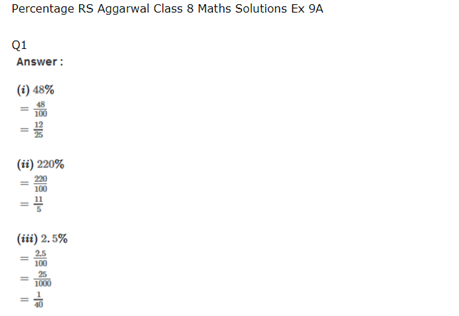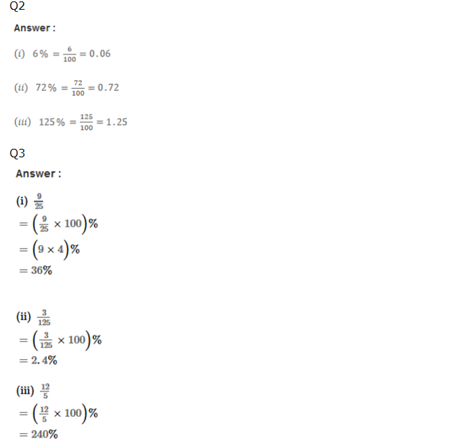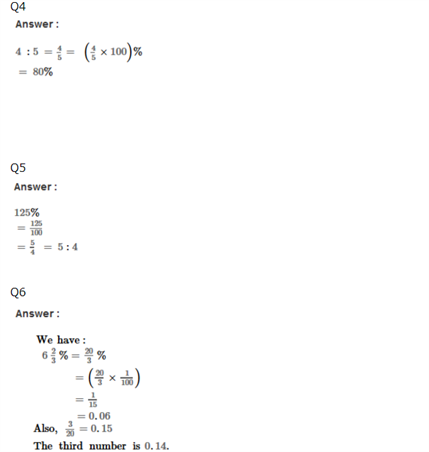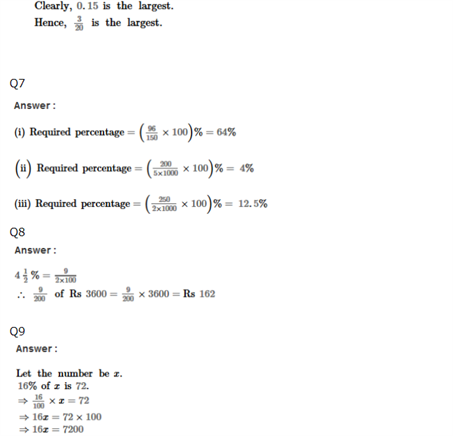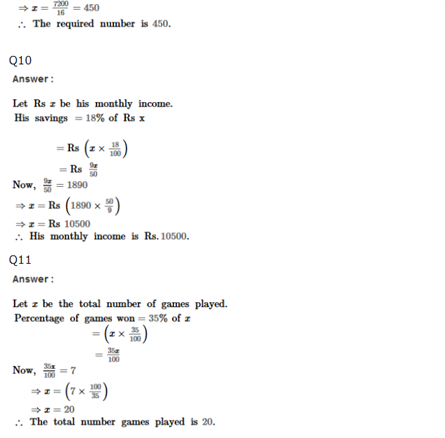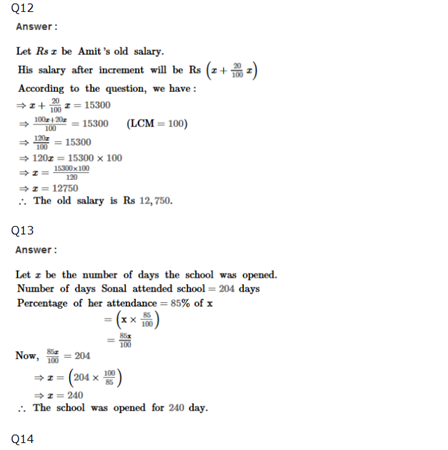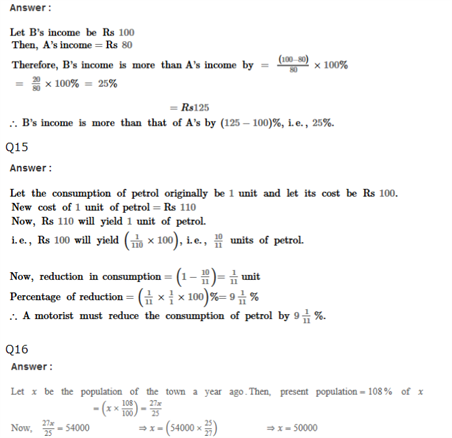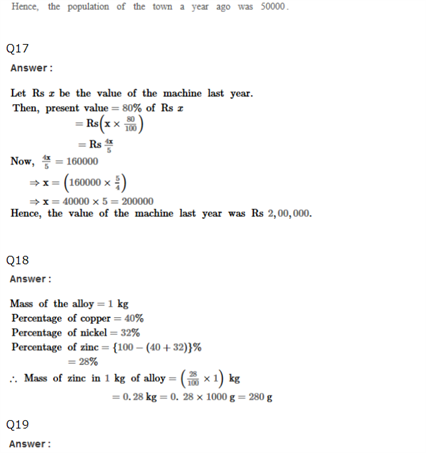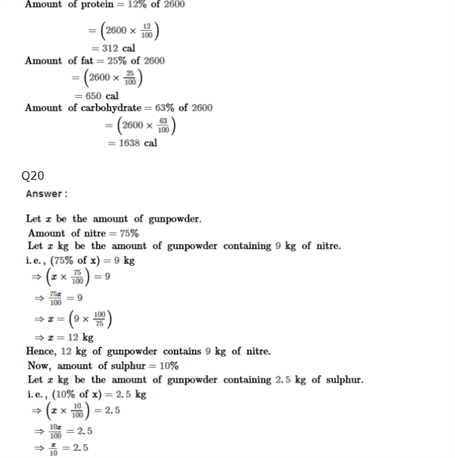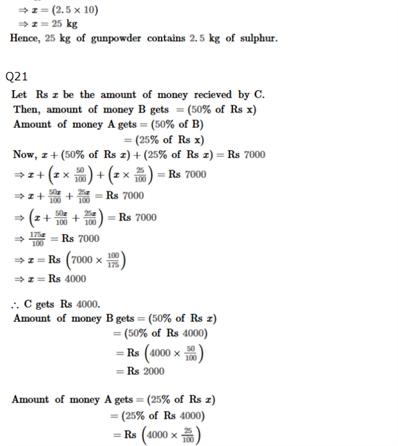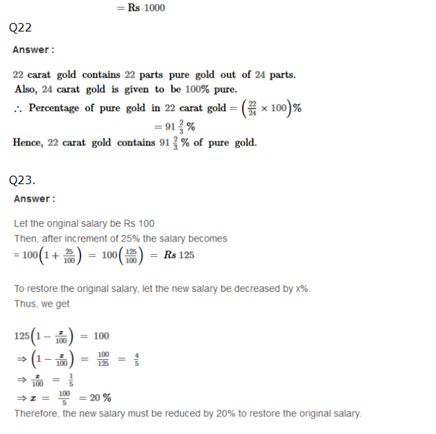Practise This Question

Subtract 86x+x27x3+x5 from x46x3+x23x+1